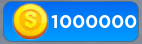# Need help with money GUI

How do i make my money on my gui show 1M instead of 1000000 like the leaderboard does.``````local cashFrame = script.Parent
local cashAmount = cashFrame.amount
local plr = game.Players.LocalPlayer

cashAmount.Text = math.ceil(plrCash.Value)

plrCash.Changed:Connect(function()

cashAmount.Text = math.ceil(plrCash.Value)
end) `````````
2 Likes

uhm you need an abbreviator, soo i cant help you with that

but i can give you an abbreviator

``````local ABBREVIATIONS = { "K", "M", "B", "T", "Qa", "Qi", "Sx","Sp","Oc","No","Dc","Ud","Dd","Td","QaD","QiD","SxD","SpD","OcD","NoD"}

local function Abbrev(num)
if num < 1000 then
end

local digits = math.floor(math.log10(num)) + 1
local index = math.min(#ABBREVIATIONS, math.floor((digits - 1) / 3))
local front = num / math.pow(10, index * 3)

return string.format("%i%s+", front, ABBREVIATIONS[index])
end
``````

and the text

``````cashAmount.Text =  Abbrev(plrCash.Value)
``````
2 Likes

You need to use something like InfiniteMath or EternityNum and use its suffix (short) function to achieve this, I am on mobile therefore I cannot provide a link however a simple search would achieve this.

This works but it doesnt save every time i rejoin it still show 1000000